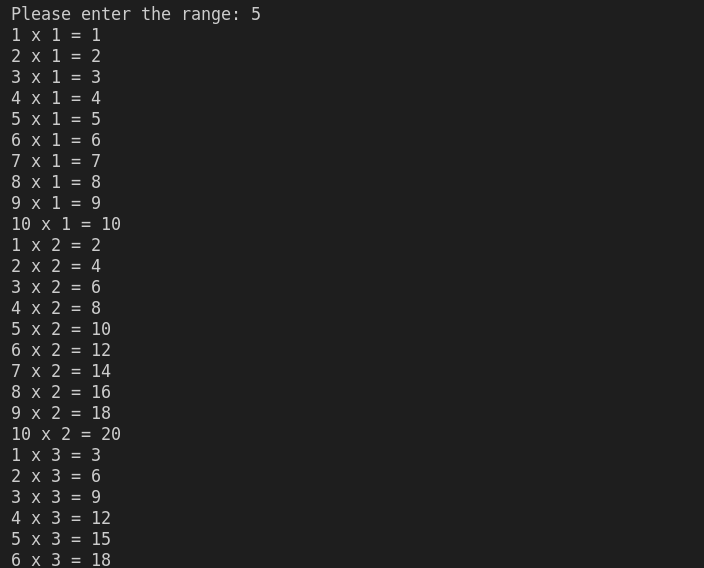Write a c++ program that takes a range from the user and then print the table to that range.

Simple Input

Sample Output

1 x 1 = 1

2 x 1 = 2

3 x 1 = 3

4 x 1 = 4

5 x 1 = 5

6 x 1 = 6

7 x 1 = 7

8 x 1 = 8

9 x 1 = 9

10 x 1 = 10

1 x 2 = 2

2 x 2 = 4

3 x 2 = 6

4 x 2 = 8

5 x 2 = 10

6 x 2 = 12

7 x 2 = 14

8 x 2 = 16

9 x 2 = 18

10 x 2 = 20

1 x 3 = 3

2 x 3 = 6

3 x 3 = 9

4 x 3 = 12

5 x 3 = 15

6 x 3 = 18

7 x 3 = 21

8 x 3 = 24

9 x 3 = 27

10 x 3 = 30

1 x 4 = 4

2 x 4 = 8

3 x 4 = 12

4 x 4 = 16

5 x 4 = 20

6 x 4 = 24

7 x 4 = 28

8 x 4 = 32

9 x 4 = 36

10 x 4 = 40

1 x 5 = 5

2 x 5 = 10

3 x 5 = 15

4 x 5 = 20

5 x 5 = 25

6 x 5 = 30

7 x 5 = 35

8 x 5 = 40

9 x 5 = 45

10 x 5 = 50

#include <iostream>
using namespace std;

int main()
{
int rang;cpp programming exercise with solution